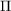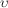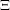International
Tables for
Crystallography
Volume G
Definition and exchange of crystallographic data
Edited by S. R. Hall and B. McMahon

International Tables for Crystallography (2006). Vol. G, ch. 2.2, p. 36

## Section 2.2.7.4.14. Greek letters

S. R. Hall,a* N. Spadaccini,c I. D. Brown,d H. J. Bernstein,e J. D. Westbrookb and B. McMahonf

#### 2.2.7.4.14. Greek letters

| top | pdf |

(31) In general, the corresponding letter of the Latin alphabet, prefixed by a backslash character. The complete set is:A \a \A alphaN \n \N nuB \b \B betaO \o \O omicronX \c \C chi\p \P pi\d \D delta\q \Q thetaE \e \E epsilonR \r \R rho\f \F phi\s \S sigma\g \G gammaT \t \T tauH \h \H etaU \u \U upsilonI \i \I iota\w \W omegaK \k \K kappa\x \X xi\l \L lambda\y \Y psiM \m \M muZ \z \Z zeta